# AP Physics C Electricity : Understanding Electrostatics

## Example Questions

### Example Question #1 : Electricity And Magnetism Exam

A hollow metal sphere with a diameter of 10cm has a net charge of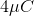distributed uniformly across its surface. What is the magnitude of the field a distance 2.0m from the center of the sphere?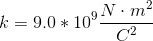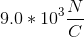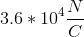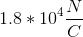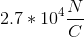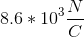Explanation:

Relevant equations: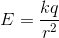(electric field of point charge)

Anywhere outside the metal sphere, the electric field is the same as it would be for a point charge of the same magnitude, located at the center of the sphere. So, calculate the electric field of a point charge given: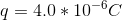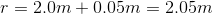Plugging in gives: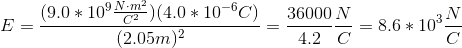### Example Question #2 : Electricity And Magnetism Exam

Two infinite parallel conducting sheets each have positive charge density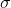. What is the magnitude and direction of the electric field to the right of the right sheet?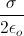, to the right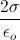, to the left, to the right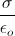, to the right, to the right

Explanation:

Relevant equations: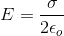(field due to single infinite plane)

Electric field is additive; in other words, the total electric field from the two planes is the sum of their individual fields: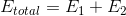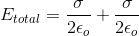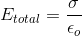The direction of the electric field is away from positive source charges. Thus, to the right of these positively charged planes, the field points away to the right.

### Example Question #1 : Electricity And Magnetism Exam

Consider a spherical capacitor made of two nested spheres. The smaller sphere has a radius of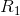and a charge of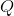, and lies within a larger sphere with radius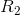and a charge of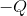Which of the following equations accurately describes the capacitance of this spherical capacitor?

Due to symmetry, this scenario would not produce capacitance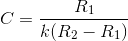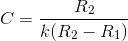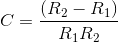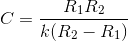Explanation:

To solve this problem, we will need to derive an equation.

We know that: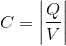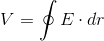We can use Gauss's law to derive the electric field between the two circles yielding: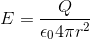Doing our integration with respect to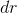fromto, we get: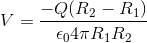We can plug this back into our equation for capacitance to get: